# 1 Goal

The goal of this tutorial is to show the differents way a time series can be forecast from the simplest method to HoltWinters method.

# 2 Preparing the data

Let’s create a simple time series with a simple seasonality and an increasing trend:

library(forecast)
ts <- ts(c(0:3,1:4,2:5,3:6,4:7,5:8,6:9), frequency = 4, start = 2011)
plot(ts)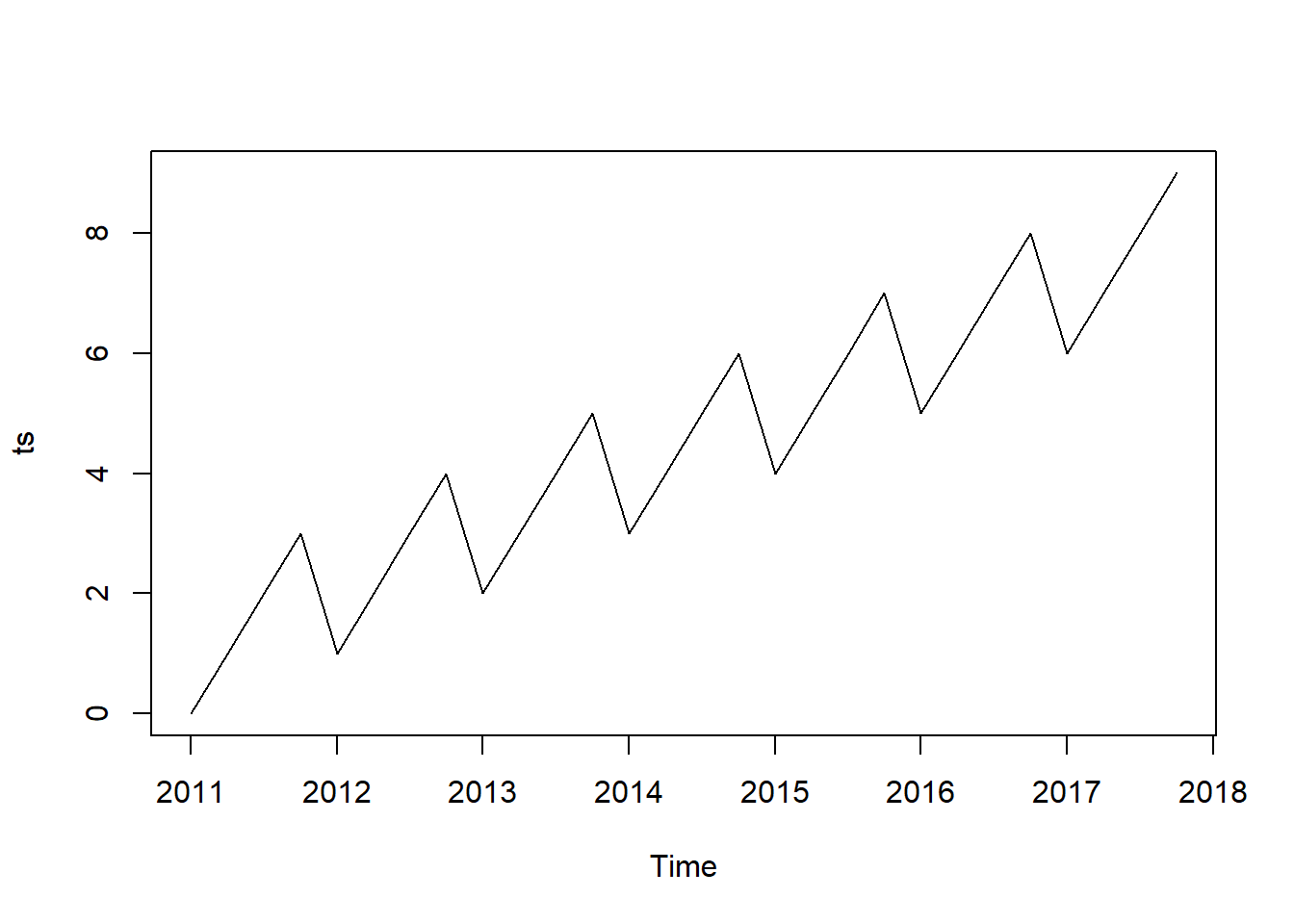The series show observations from 2010 to 2017 with a frequency of 4 observations per year.

# 3 Forecasting

Now let’s forecast the next two years values through average method:

## 3.1 Average method

# Average method
plot(meanf(ts, h = 8))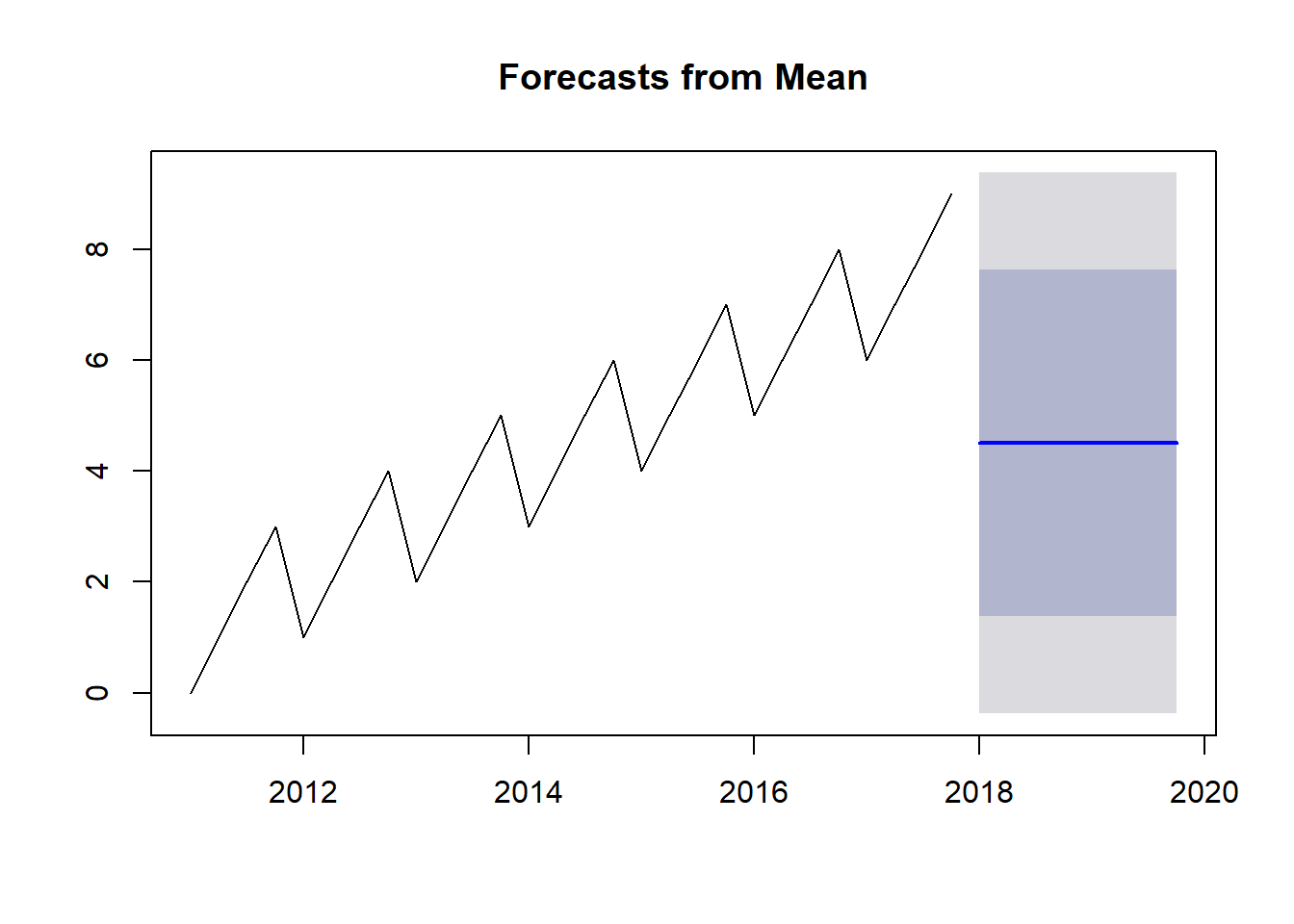As we can see, forecasting using the mean of all the values on the time series does not seem a realistic prediction. So, now we will use the naïve method, which forecasts the next values using the last value observed.

## 3.2 Naïve method

# Naive method
plot(naive(ts, h = 8))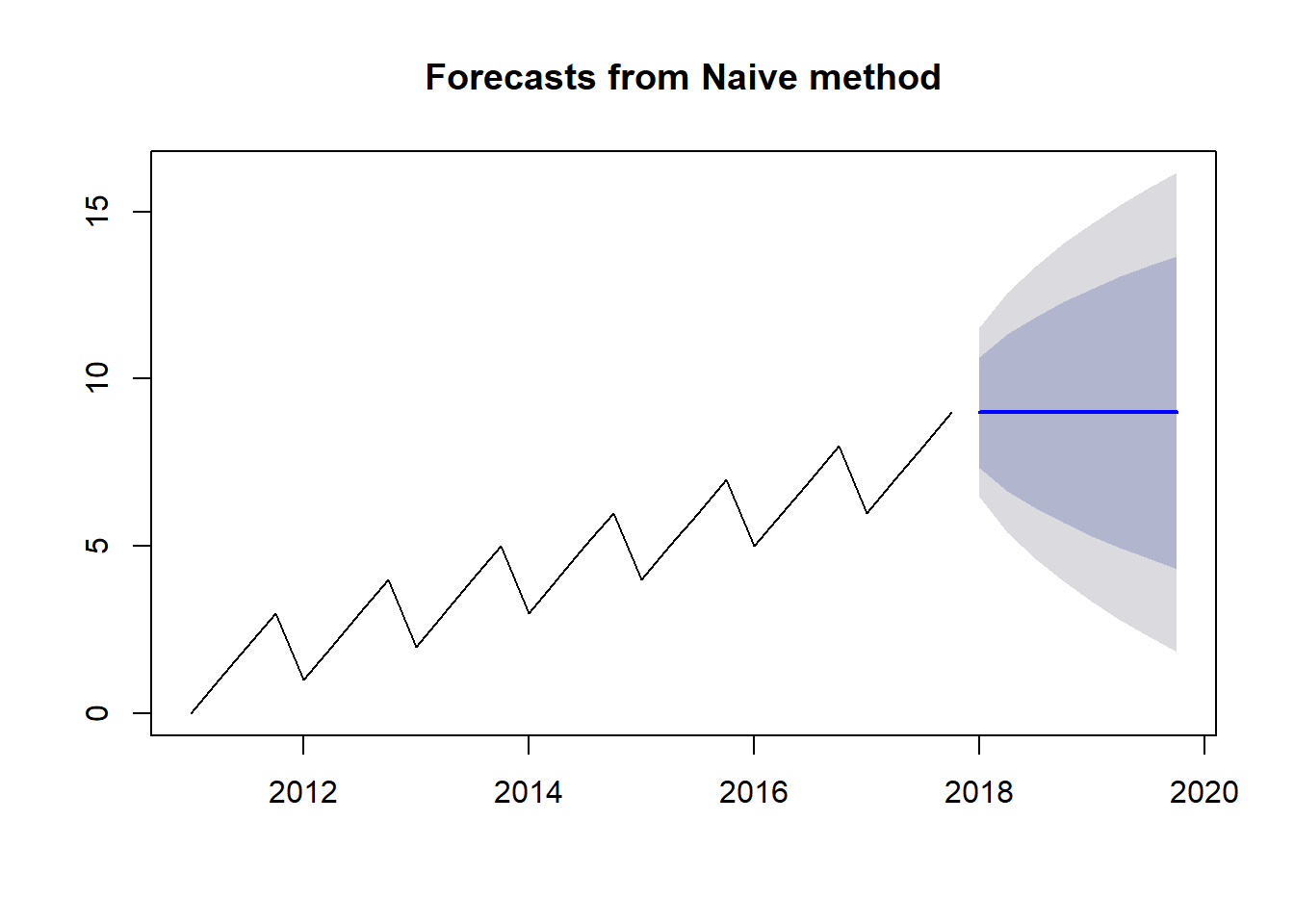Now the prediction seems more accurate than the average method, but this prediction is not taking into account the seasonality of the serie nor the trend. The seasonality pattern can be shown with the season naïve method, which will take the values of the last cycle.

## 3.3 Seasonal naïve method

## Seasonal Naïve method
plot(snaive(ts, h = 8))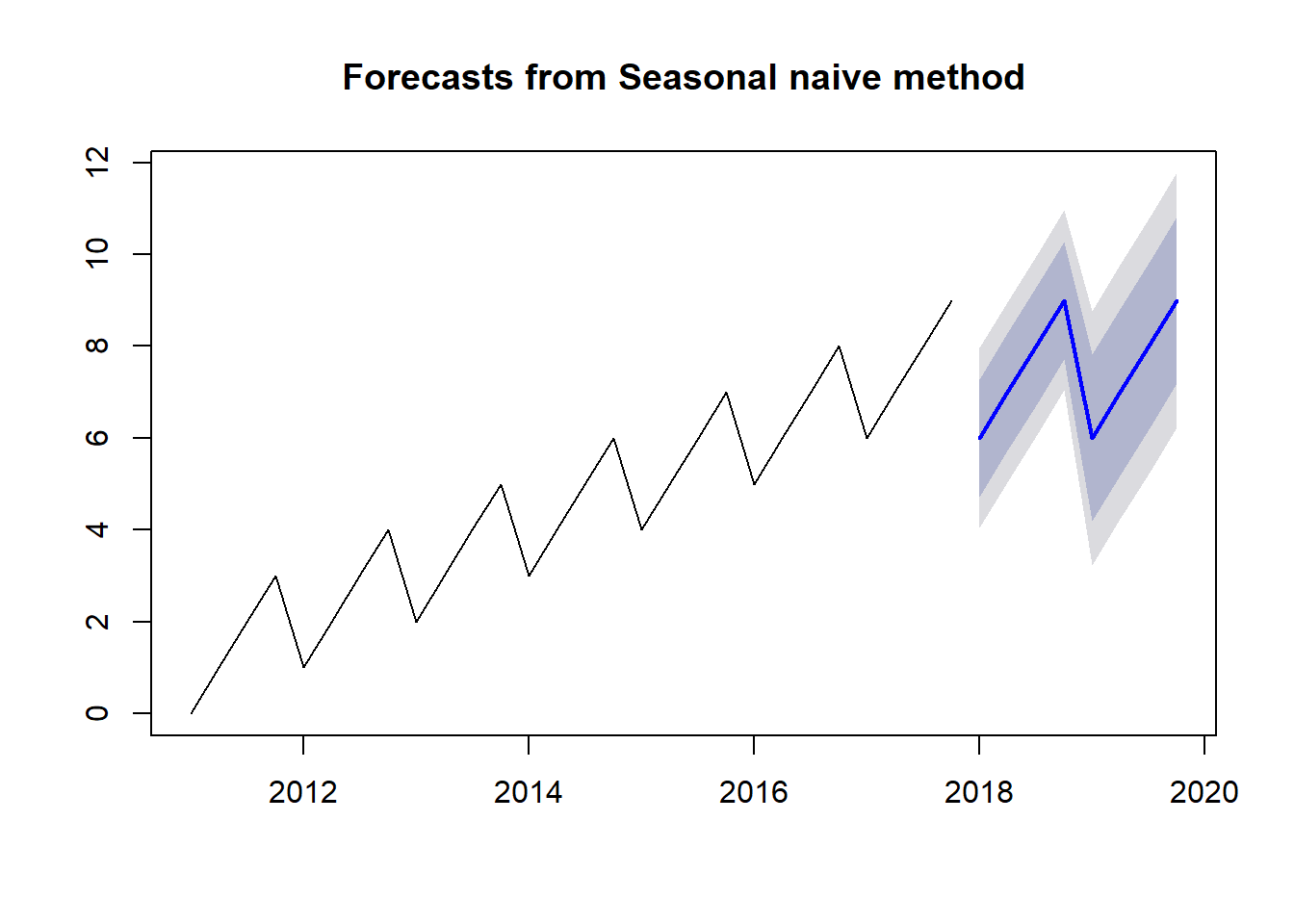The seasonal naïve method is using the value from the first quarter of 2017 in order to predict the first quarter of 2018. The prediction of the second quarter of 2018 is done with the value of the second quarter of 2017, and so on. Nevertheless, although this method is more accurate and complex that the methods shown before, the trend has not been recognized yet.

The simplest method to predict using the general trend of the serie is the drift method, which prediction will be the result of drawing a line between the first and the last line of the serie.

## 3.4 Drift method

# Drift method
plot(rwf(ts, h = 8, drift = T))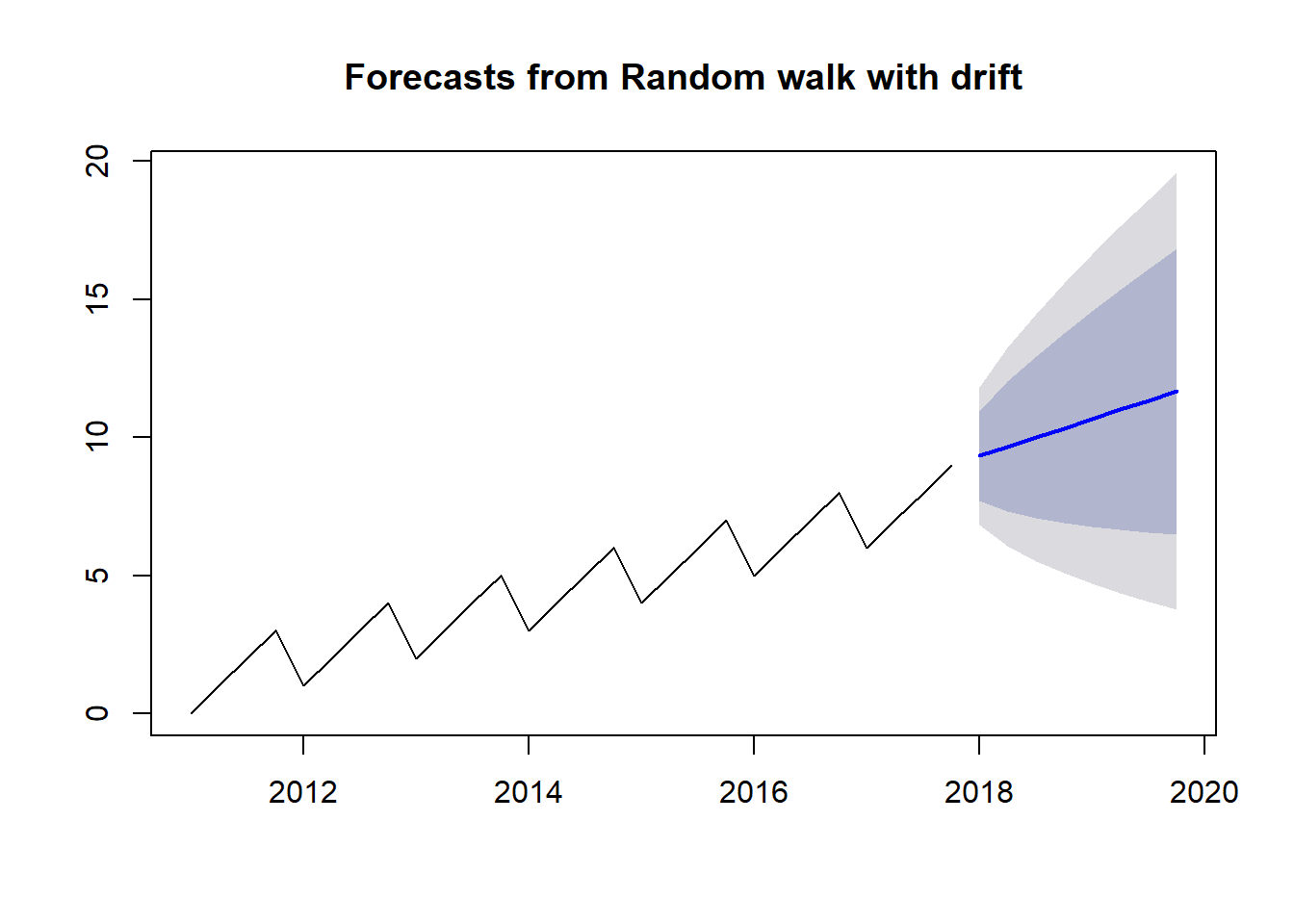This prediction understands the trend but lacks the seasonality pattern.

Finally, we will use the HoltWinters forecast method, which will combine the season naïve and drift methods.

## 3.5 Holt Winters forecast method

# Holt Winters forecast
plot(forecast(HoltWinters(ts), h = 8))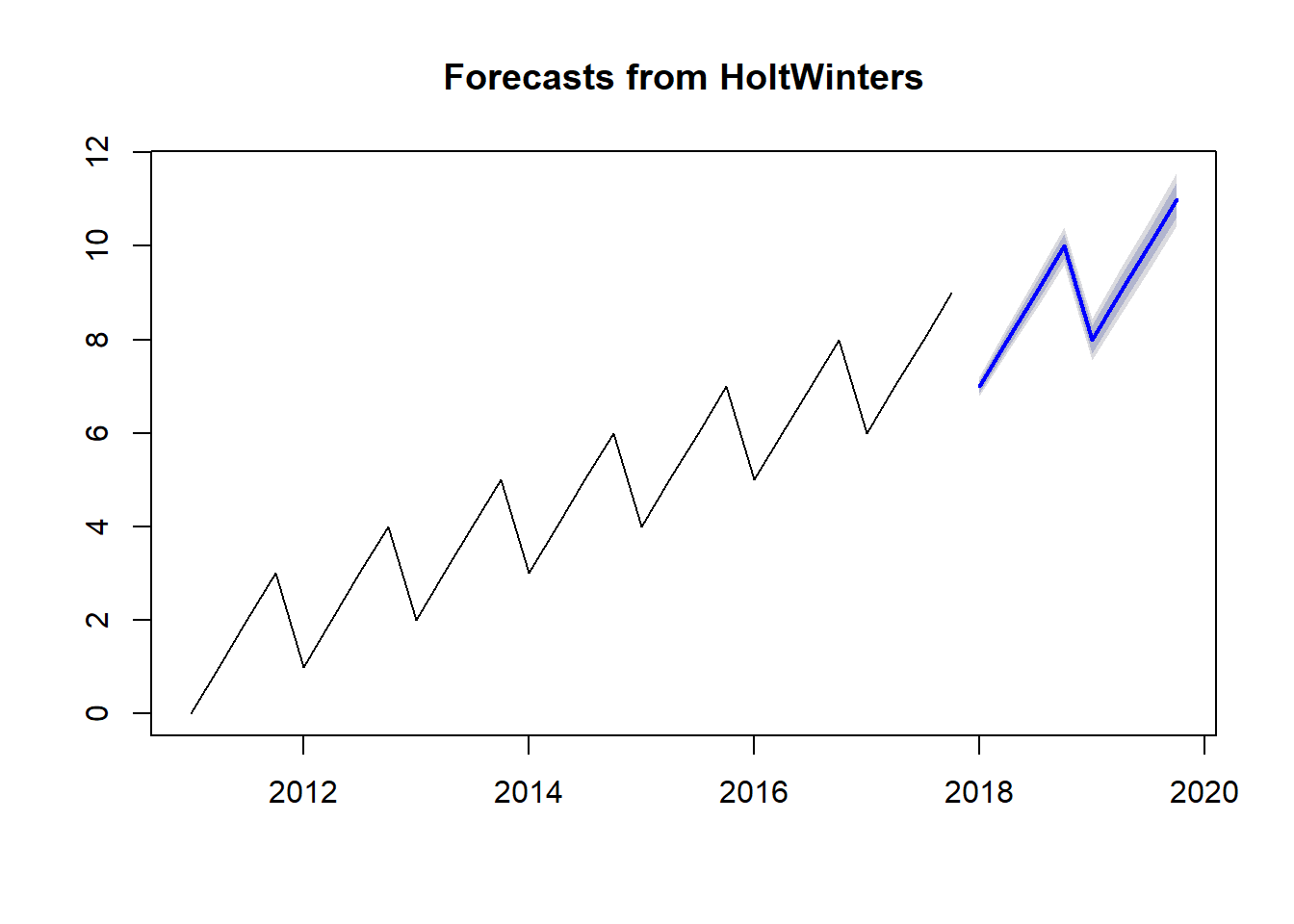The results of the forecast show that the prediction fits perfectly the trend and the seasonality of our time series.

# 4 Conclusion

We have learnt through this tutorial to understand the different methods used to forecast a time series and how Holt Winters combine them in order to fit the prediction with the time series patterns.